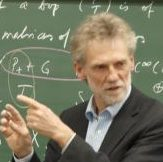Elmar Schrohe
(Leibniz Universität Hannover)
January 12, 2021
15:00
(CET)
Organized by Europe GNCG Seminar.

## Index Theory for Fourier Integral Operators and the Connes-Moscovici Local Index Formulae

The index theory for operator algebras generated by pseudodifferential operators and Fourier integral operators, more specifically Lie groups of quantized canonical transformations, has attracted a lot of attention over the past years. It can be seen as a universal receptacle for a wide range of index problems such as the classical Atiyah-Singer index theorem, the Atiyah-Weinstein problem, or the B ̈ar-Strohmaier index theory for Dirac operators on Lorentzian spacetimes. It also includes work by Connes-Moscovici, Gorokhovsky-de Kleijn-Nest, or Perrot.

In my talk, I will focus on the particularly transparent situation, where the pseu-dodifferential operators are Shubin type operators on euclidean space. We first study the case, where the Fourier integral operators are given by metaplectic oper-ators, then we add a Heisenberg type group of translations, so that we obtain the quantizations of isometric affine canonical transformations.

We find a cohomological index formula in the first case. In the second, our algebra encompasses noncommutative tori and toric orbifolds. We introduce a spectral triple (A, H, D) with simple dimension spectrum. Here H = L2(Rn, Λ(Rn)) and D is the Euler operator. a first order differential operator of index 1. We obtain explicit algebraic expressions for the Connes-Moscovici cyclic cocycle and local index formulae for noncommutative tori and toric orbifolds.

Joint work with Anton Savin (RUDN, Moscow).